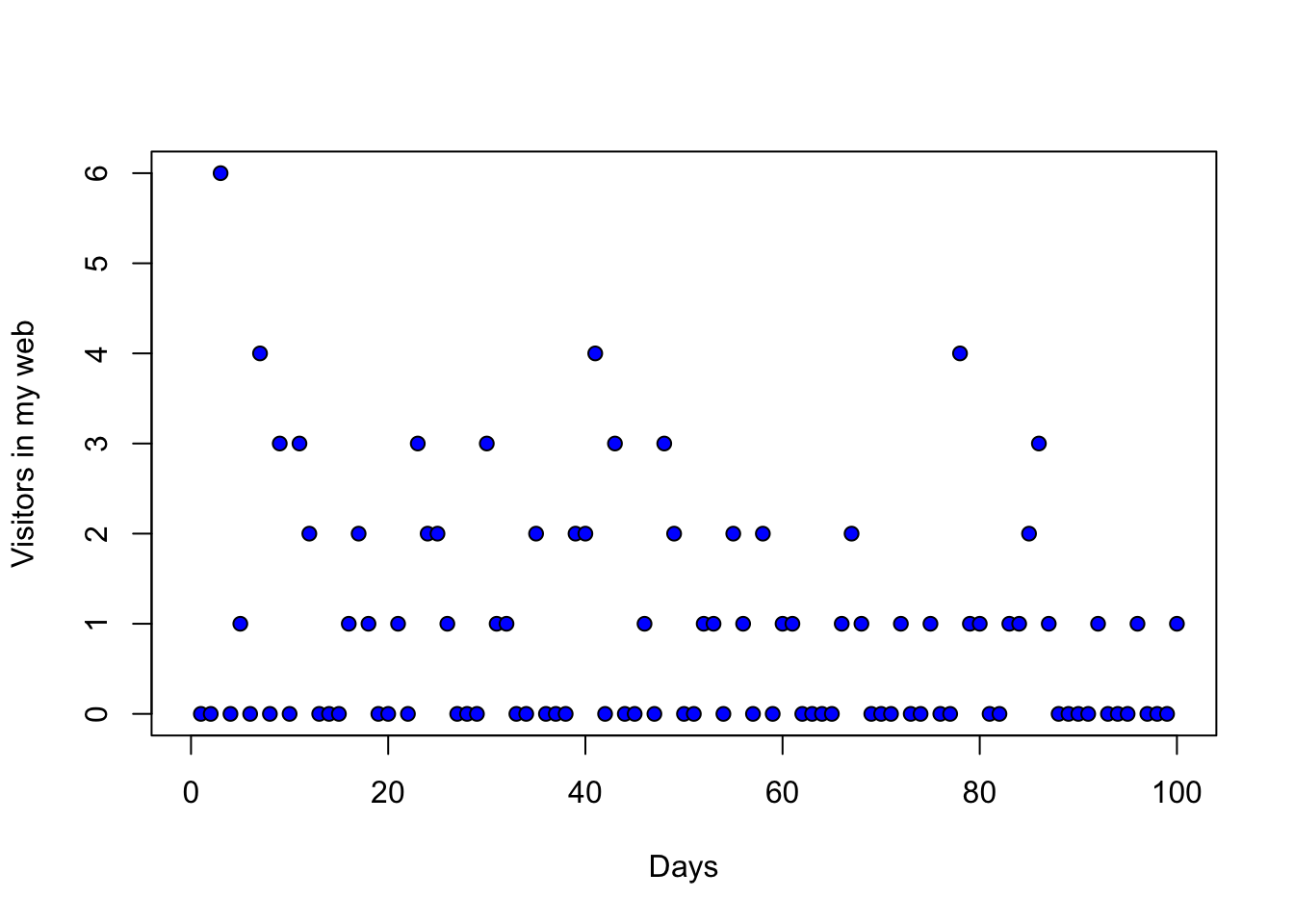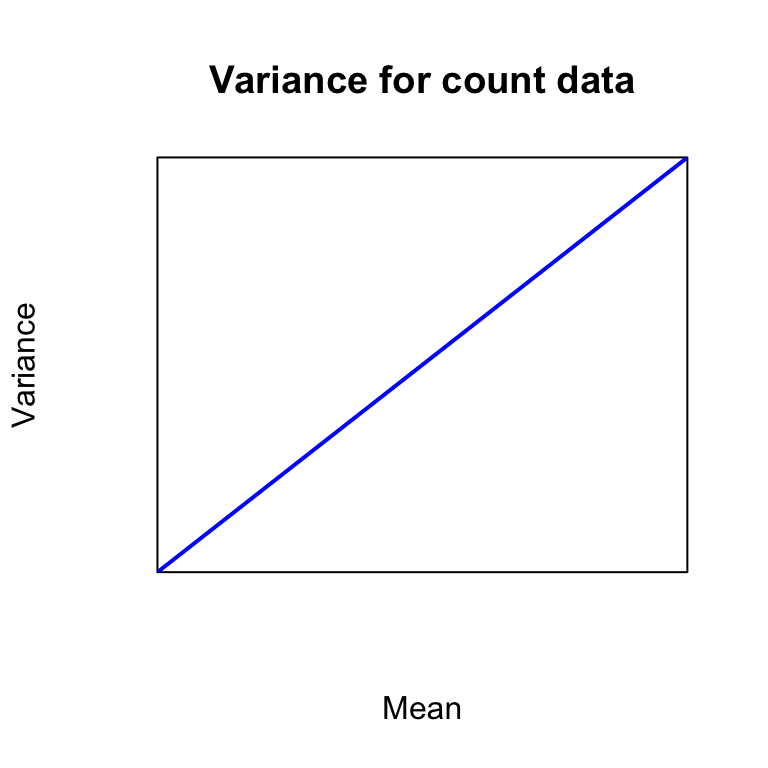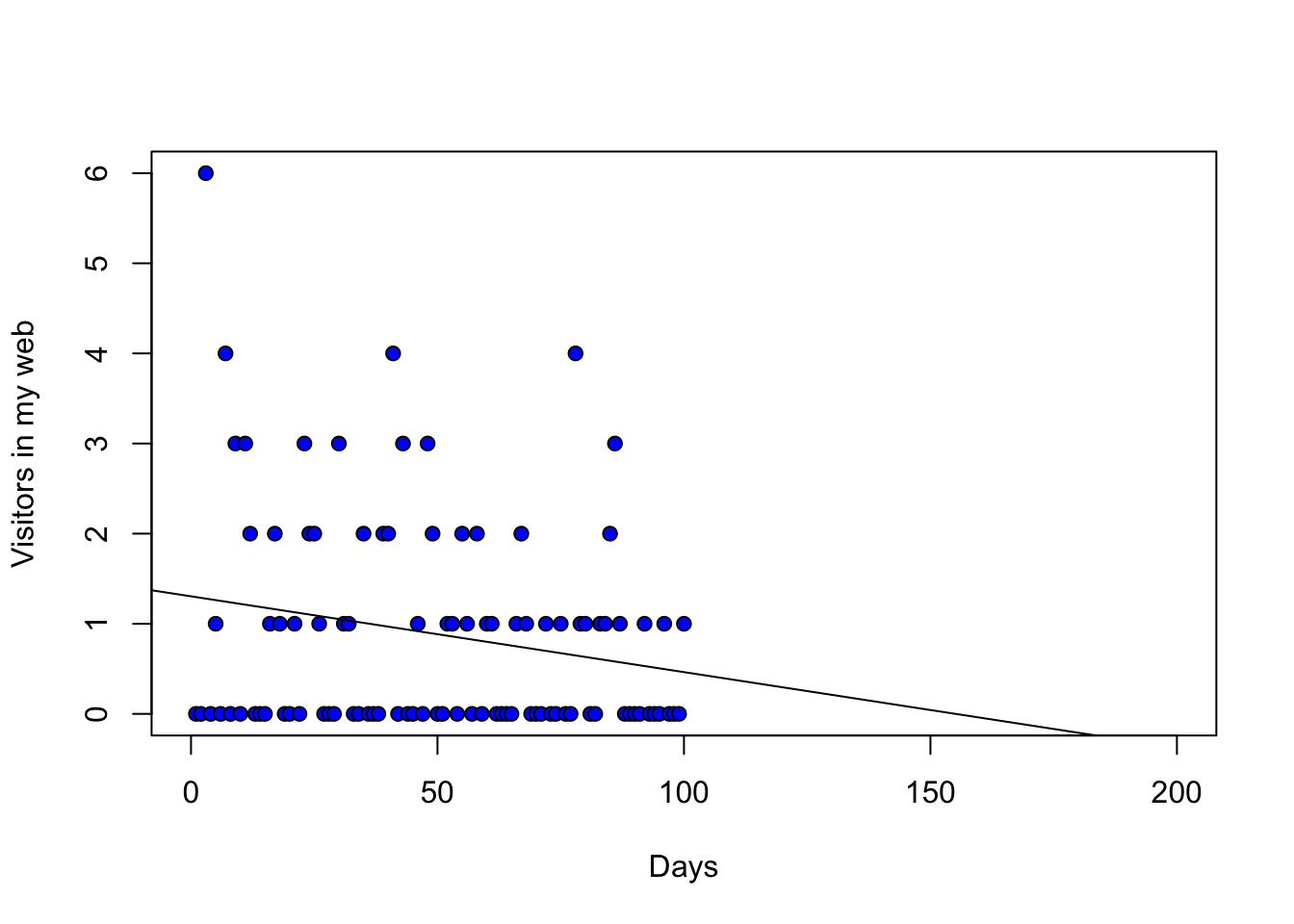NOTE

This text serves as notes of the Research Methods in Forest Sciences 2017 class (30/10/2017). It is not a book or any official document, and although it has been created to somehow being useful it comes with absolutely no warranty of any sort. As any work in progress you might find incomplete information and mistakes. I welcome constructive criticism and new ideas.

## Introduction

Until now you have explored cases where the response variable has been a continuous real number (e.g. height, weight, length,…), and you have learnt how those variables can be modeled using a linear model. When we modeled continuous response variables using linear models we made several important assumptions about its behaviors:

• constant variance
• normal errors
• independent errors
• random sampling

In real life, many times we will also work with other response variables like frequencies or counts, in those cases the response it is not anymore a continuous variable. These response variables violate some of the assumptions that we made when using linear models. Some of these response variables are:

• count data
• proportion data
• binary response data
• survival data (age-at-death data)

All of these responses variables fail the assumptions about constancy of variance and normality of errors.

Here we are going to discuss why we can not use linear models when the response variables are frequencies or counts and what is then the best aproach to model them.

## Count data

Count data is data on frequencies, we count how many times something happened (whole numbers or integers). For example:

• Number of dead trees after storm
• Number of people visiting my web page per day
• Number of red blood cells on a microscope slide

In these cases we know the number of times that something happen, but not the number of times it did not happen. For example I know the number of visitors in my web but I do not know the number of people that did not visit my web and was online on the same day.

If we use the second example: “Number of people visiting my web page per day”:

Response variable (count): the number of reported visitors per day in my web

Explanatory variable: the number of days since my web is online

This is the graph plotting the data:### Variance in count data

When the amount of counts is low (e.g. many days I get 0 visitors, and other days I get 1 or 2 visitors) the mean number of visitors across the 100 days is going to be low. When the mean is low the variance is also low (remember that variance is the sum of the squares of the departures of the counts from the mean count, divided by the degrees of freedom). But when the mean is high, for example if the number visitors in my web vary between 0 and 50 across 100 days having many of the days more than 20 visitors; when I calculate the residuals in this case and square them we can expect to obtain very large numbers, and a consequently high variance. This means that for count data the variance is expected to increase with the mean, rather than being constant (as assumed in linear models).Problem 1: Count data variance is expected to increase with the mean, rather than being constant.

### Problems of modelling count data with a linear model

Lets now try to answer the question: Does time (number of days since I published my web) affects the number of web visitors?

The first thing we can do is to look at the data plot (above). As you can see the values of the response “number of visitors in my web”, are dispersed in discrete rows and don’t cluster around a regression line. You can also notice that there are a lot of zeros, many of the days I did not get any visitor in the web. There seems to be a negative trend in the data, as more days passed by I had less visitors, but is the trend significant?

If I try to obtain a linear model using R I obtain this summary model output:

``````##
## Call:
## lm(formula = webvisitors\$nvisitors ~ webvisitors\$days)
##
## Residuals:
##     Min      1Q  Median      3Q     Max
## -1.2964 -0.8779 -0.4846  0.4775  4.7204
##
## Coefficients:
##                   Estimate Std. Error t value Pr(>|t|)
## (Intercept)       1.304848   0.237962   5.483 3.25e-07 ***
## webvisitors\$days -0.008413   0.004091  -2.056   0.0424 *
## ---
## Signif. codes:  0 '***' 0.001 '**' 0.01 '*' 0.05 '.' 0.1 ' ' 1
##
## Residual standard error: 1.181 on 98 degrees of freedom
## Multiple R-squared:  0.04137,    Adjusted R-squared:  0.03159
## F-statistic: 4.229 on 1 and 98 DF,  p-value: 0.0424``````The black line is the linear model that I obtained. If you pay attention you will notice that the linear model (black line) is predicting that after approx. 170 days of my web being online I will have negative visitors! Count data are strictly bounded below, I can not have negative visitors in my web, I can only have 0 or more visitors.

Problem 2: The response is a count and thus is non-negative, but our linear model doesn’t acknowledge that.

If we now have a look to the residuals using the linear model: